## Spatial data

Lots of data in our field(s) are inherently spatial

R has lots of tools for interacting with and analyzing spatial data

## the `sp` package

The `sp` package is the workhorse of spatial mapping and analysis in R.

It defines spatial classes (e.g. `SpatialPoints`, `SpatialPointsDataFrame`) that make it possible to work with spatial data.

## actual spatial data versus coordinates

We often just have coordinate data, but this works differently in R from fully spatial data

```myCoords <- data.frame(long = runif(20, min=35, max=36), lat = runif(20, min = 3, max=5))
plot(myCoords)```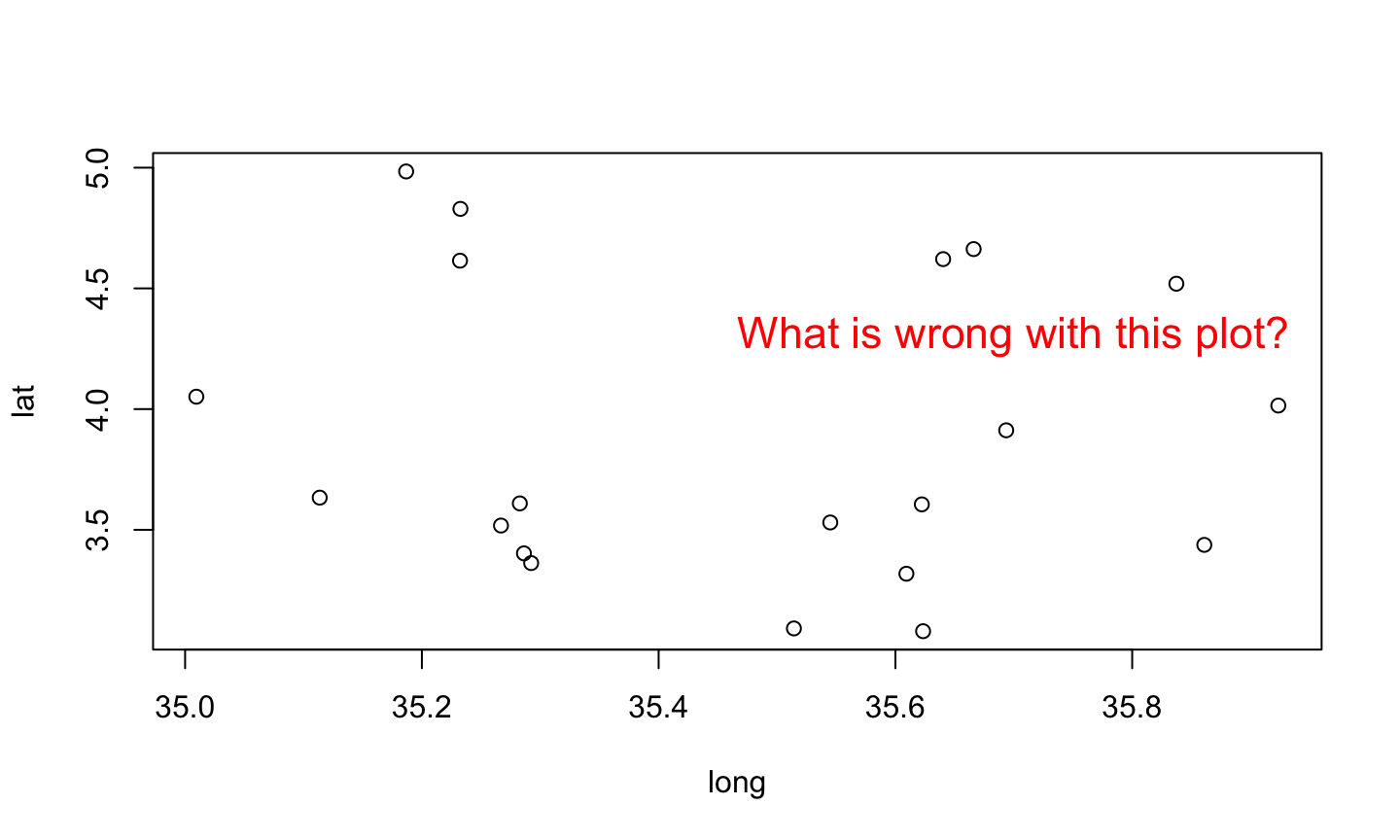## making spatial objects

You can use the `SpatialPoints()` function to make your coordinates into a fully spatial object

Warning: This function will assume that your columns are in the order: X coordinate, Y Coordinate

For a gold star: Which is the x and which is the y when we are dealing with latitude and longitude?

```library(sp)
spMyCoords <- SpatialPoints(coords = myCoords)```

Now there are a plethora of spatial things you can do with these points that you couldn't before

`bbox(spMyCoords)`
```##            min       max
## long 35.009496 35.923433
## lat   3.079992  4.984301```

## making spatial objects

`plot(spMyCoords, axes=TRUE)`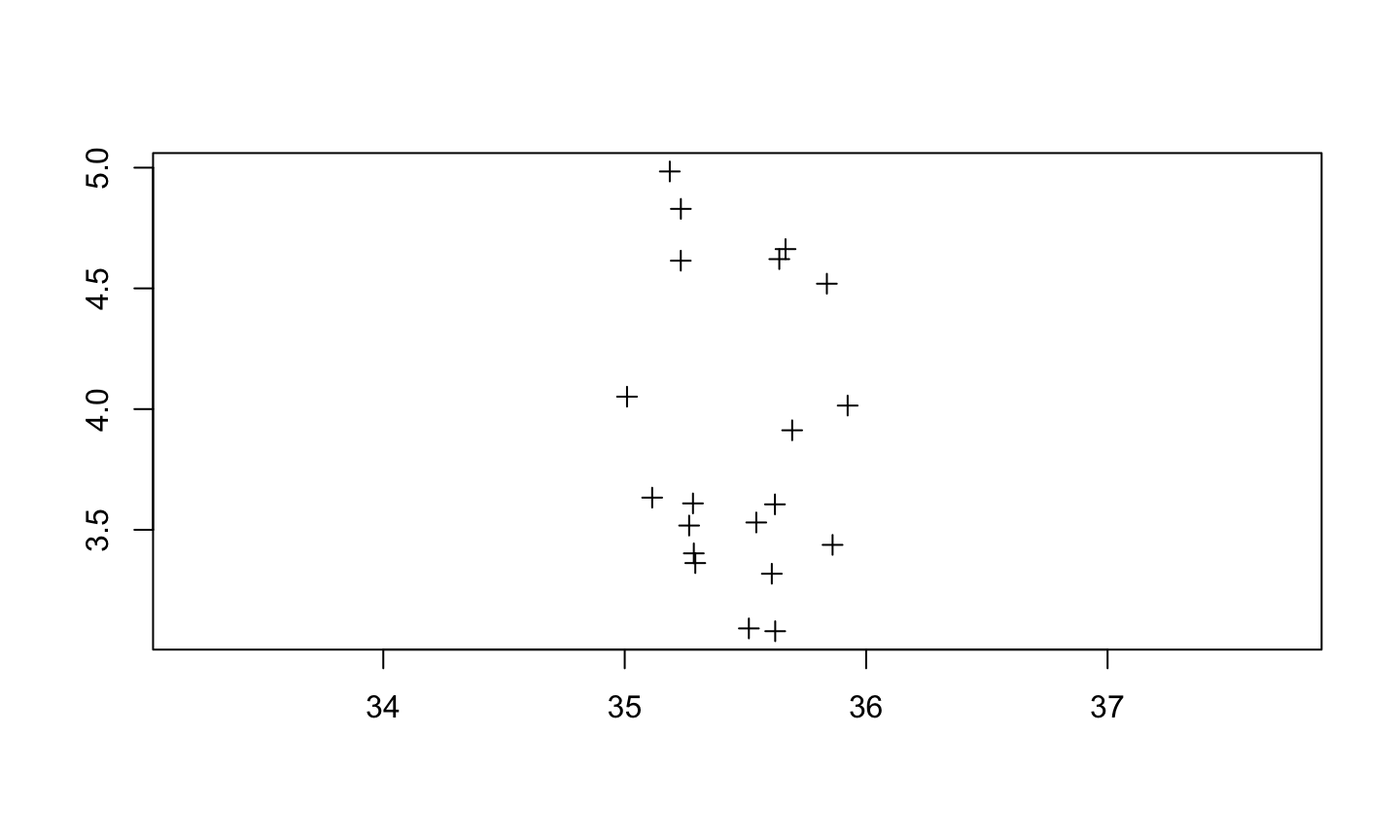## maps

The `maps` package provides basic maps that can serve as a backdrop to your points

```library(maps)
world <- map(database = "world")```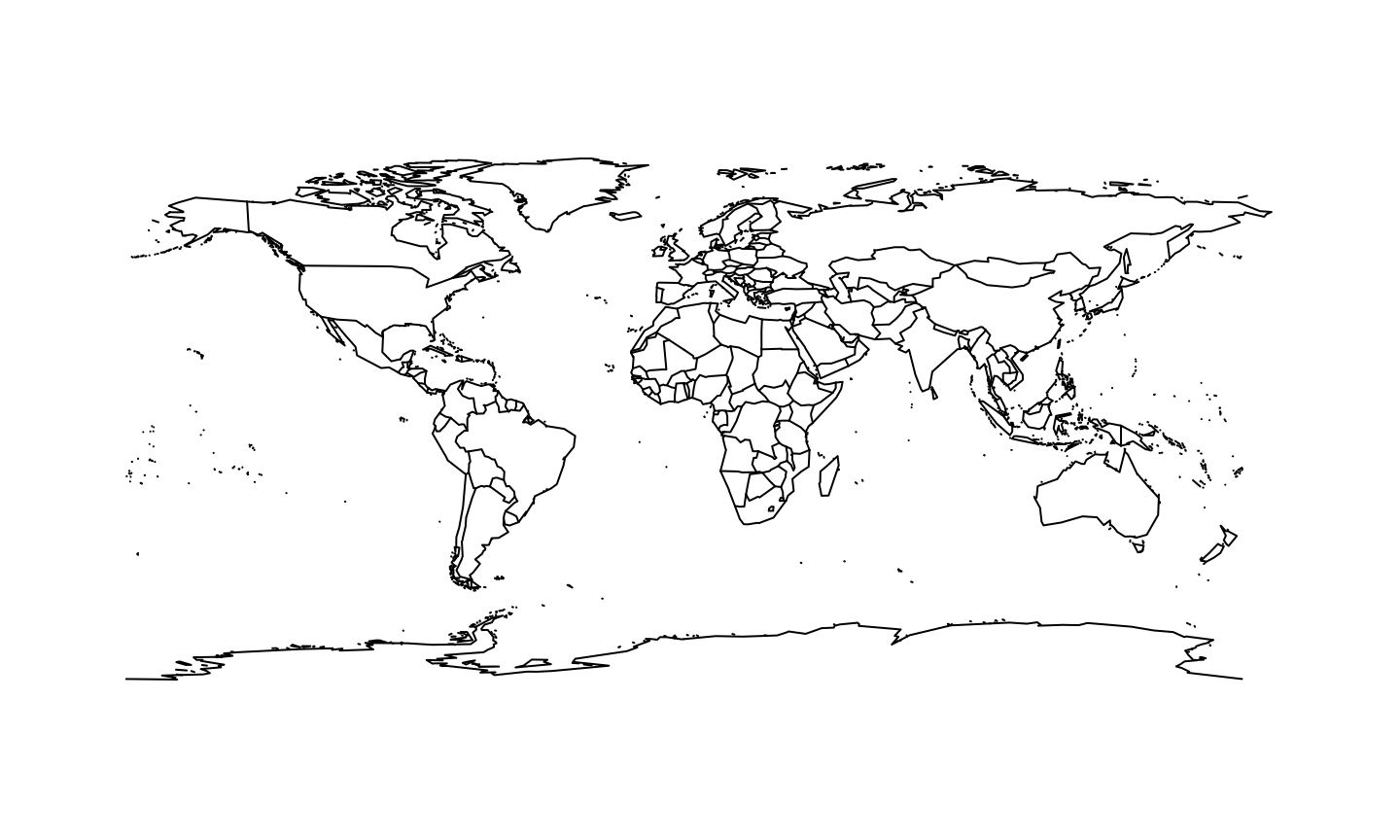## maps

### This is not an accurate election map!

`USA <- map(database="state", fill=TRUE, col=c("red","blue"))`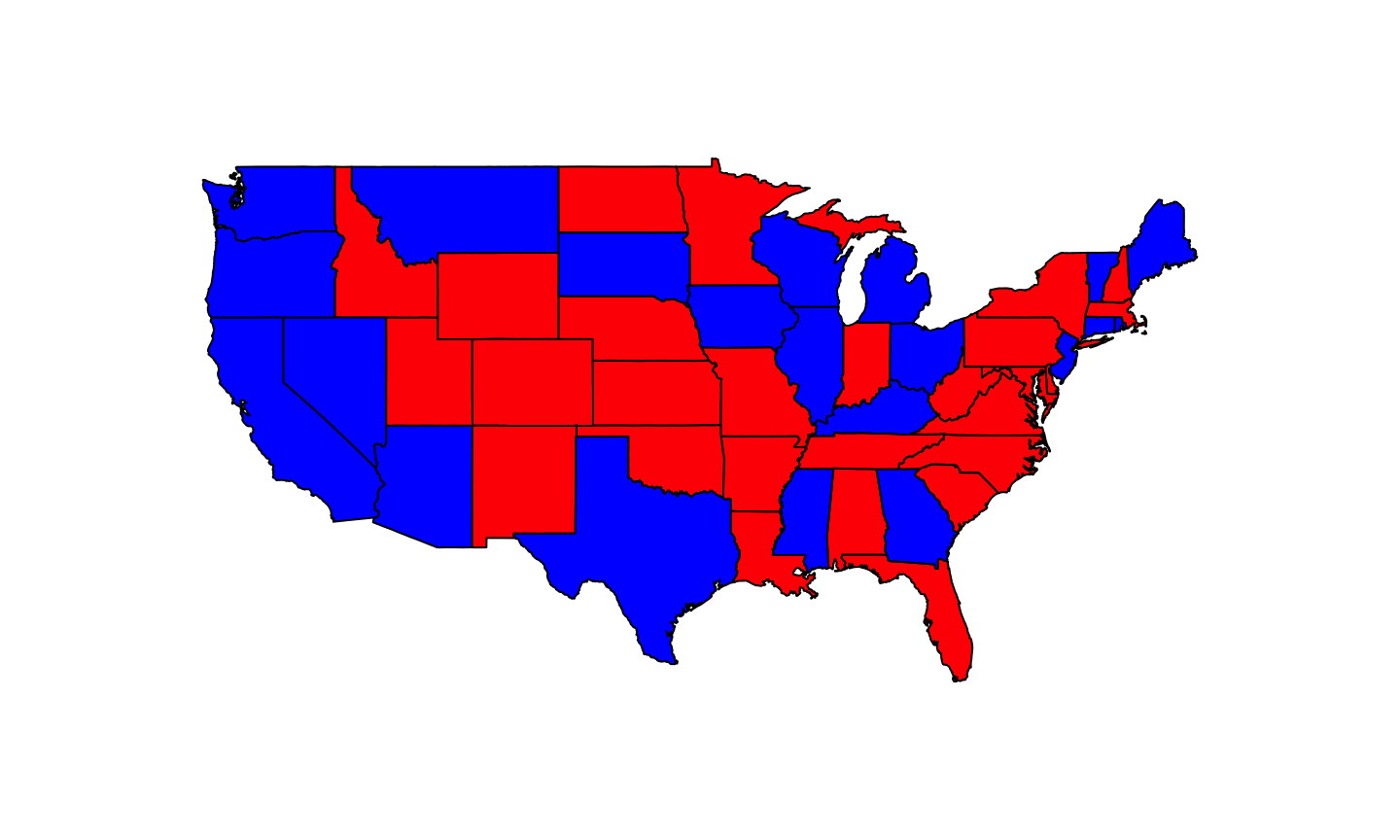## maps

`kenya <- map(database = "world", region="Kenya")`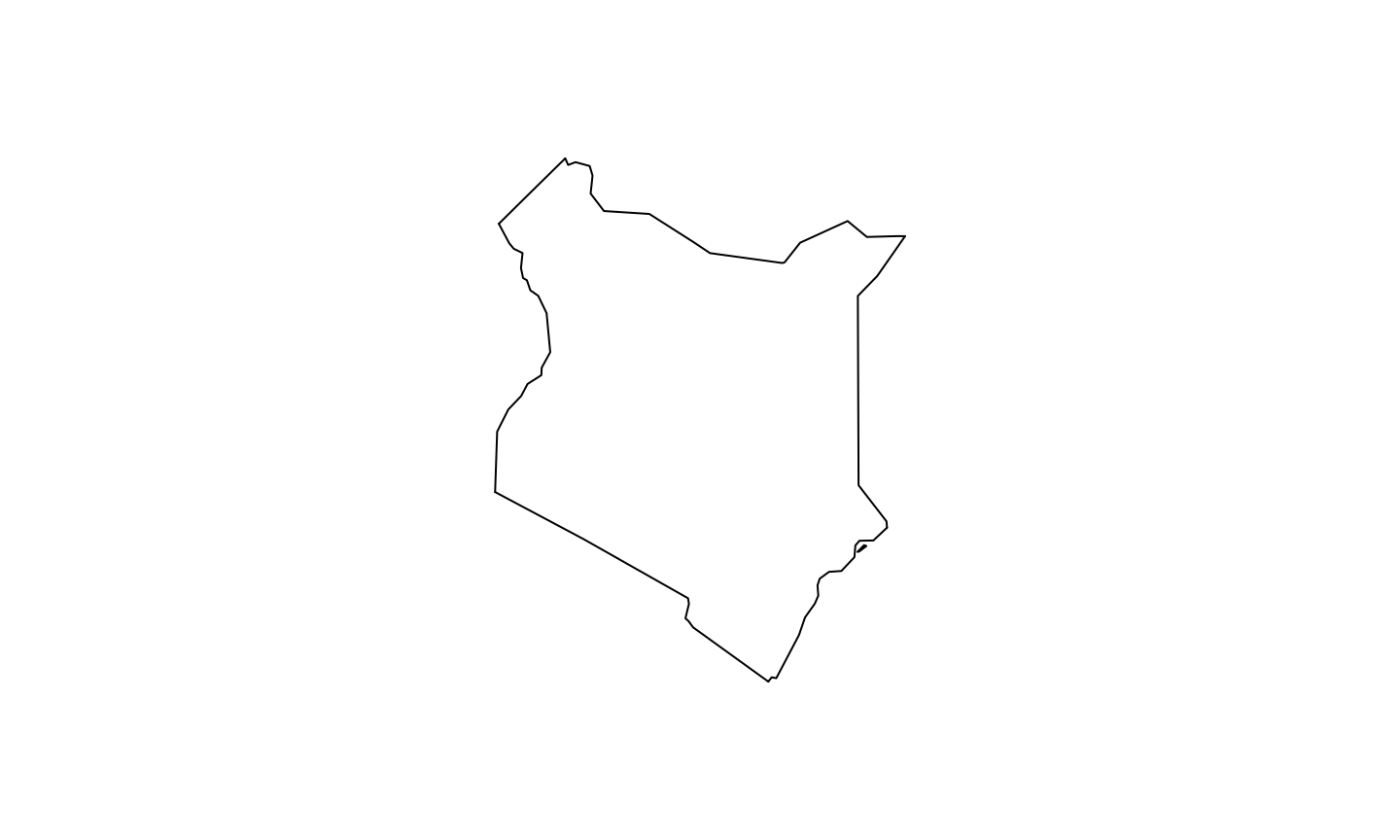## Challenge

Plot the `spCoords` points as solid blue filled circles on top of the Kenya map

```library("RgoogleMaps")